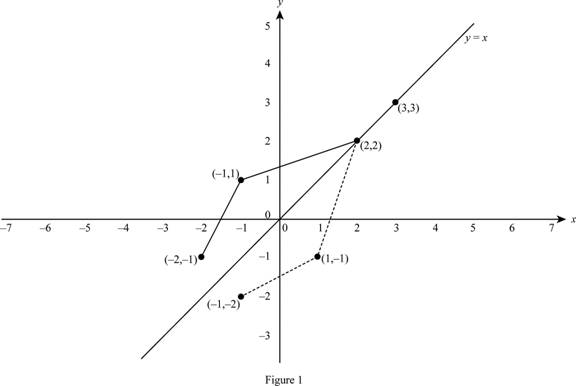# The graph of f − 1 from the given graph of f .### Single Variable Calculus: Concepts...

4th Edition
James Stewart
Publisher: Cengage Learning
ISBN: 9781337687805### Single Variable Calculus: Concepts...

4th Edition
James Stewart
Publisher: Cengage Learning
ISBN: 9781337687805

#### Solutions

Chapter 1.6, Problem 29E
To determine

## To sketch: The graph of f−1 from the given graph of f.

Expert Solution

### Explanation of Solution

Identify the points from the given graph.

The points plotted in the graph are (−1, −2), (1, −1), (2, 2) and (3, 3).

Interchange the x and y values to find the points of the inverse graph.

Thus, the inverse function contains the points, (−2, −1), (−1, 1), (2, 2) and (3, 3).

Recall the fact that the inverse function is the reflection about the line y=x.

So, to sketch the graph of f1, draw the line y=x  and plot the points (−2, −1), (−1, 1), (2, 2) and (3, 3).

Thus, the graph of f1 is shown below in Figure 1.From Figure 1, it is observed that the graph of f1 is the reflection of the graph of f about the line y=x.

### Have a homework question?

Subscribe to bartleby learn! Ask subject matter experts 30 homework questions each month. Plus, you’ll have access to millions of step-by-step textbook answers!